Pool

If water flows into the pool by two inlets, fill the whole for 18 hours. The first inlet filled pool 6 hour longer than second. How long pool take to fill with two inlets separately?

Result

t1 =  39.25 h
t2 =  33.25 h

Solution:Checkout calculation with our calculator of quadratic equations.Leave us a comment of example and its solution (i.e. if it is still somewhat unclear...):Math student
1/t1+1/(t1-10)=1/18
multiply each term by18(t1)(t1-10)
that results in
18(t1-10)+18t1=t1(t1)(t1)-10t1
using the quadratic formula results in t1=-49.6 and 3.63Dr Math
right side of equation is wrong - should be t1*(t1-10) = t12 - 10*t1 now t13-10t1Math student
the problems seems to have changed - - - t2 is now equal t1-6

therefore 1/t1+1/(t1-6)=1/18
multiplying each term by18(t1)(t1-6) ==== 18(t1-6)+18t1=t1(t1-6), simplifying further 18t1-108+18t1=t12-6t1
or 0=t12-6t1-18t1+108
graphing y=18(t1-6)+18t1-t1(t1-6) results in t1=39.25 hours and t2=39.25-6=33.25 hours (same as your NEW answer!!!!To solve this example are needed these knowledge from mathematics:

Looking for help with calculating harmonic mean? Looking for a statistical calculator? Looking for help with calculating roots of a quadratic equation? Do you have a linear equation or system of equations and looking for its solution? Or do you have quadratic equation? Tip: Our volume units converter will help you with converion of volume units.

Next similar examples:

1. Tank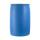In the middle of a cylindrical tank with a bottom diameter 251 cm is standing rod which is 13 cm above the water surface. If we bank rod its end reach surface of the water just by the tank wall. How deep is the tank?
2. Factors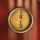Can the expression ? be factored into rational factors?
3. Rectangular cuboidThe rectangular cuboid has a surface area 5334 cm2, its dimensions are in the ratio 2:4:5. Find the volume of this rectangular cuboid.
4. CuboidCuboid with edge a=16 cm and body diagonal u=45 cm has volume V=11840 cm3. Calculate the length of the other edges.
5. Rhombus and inscribed circleIt is given a rhombus with side a = 6 cm and the radius of the inscribed circle r = 2 cm. Calculate the length of its two diagonals.
6. TangentsTo circle with a radius of 41 cm from the point R guided two tangents. The distance of both points of contact is 16 cm. Calculate the distance from point R and circle centre.
7. Trolleybus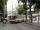Trolleybus line No. 206 measured 24 km. If the trolleybus goes faster by 5 km/h, the way there and back would is shorter by 33 minutes. Calculate the trolleybus speed and how much time it takes a return trip.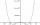By introducing a new variable solve biquadratic equation: ?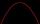Solve pure quadratic equation ?.Solve quadratic equation: 2x2-58x+396=0
11. SquareIf the length of the sides of the square we decrease by 25% decrease the content area of 28 cm2. Determine the side length of the original square.
12. 3d vector componentThe vector u = (3.9, u3) and the length of the vector u is 12. What is is u3?
13. Apples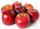A 2 kg of apples cost a certain sum of money. This sum is equal to the amount of kilograms for which we pay 72 CZK. How much is 1 kg of apples?
14. ProductThe product of two consecutive odd numbers is 8463. What are this numbers?
15. Trapezoid 15Area of trapezoid is 266. What value is x if bases b1 is 2x-3, b2 is 2x+1 and height h is x+4
16. TrapeziumThe length of the base and the height size of the base of the trapezium is at ratio 5:3:2, the content area of the trapezium is 128 cm2. Calculate the length of the base and the height of the trapezoid.
17. Diagonal of the rectangleCalculate the diagonal of the rectangle which area is 54 centimeters square and the circuit is equal to 30 cm.